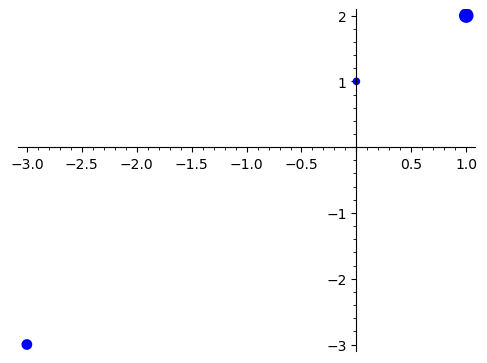# Revision history [back]

Using point2d is indeed the right idea.

We will need to

• scale the values of M to turn them into reasonable point sizes for point2d.
• use a separate point2d command for each point size

Here is an example using the values in the question:

Define M as a dictionary:

sage: M = {(0, 1): 0.3, (1, 2): 1.1, (-3, -3): 0.6}


Plot:

sage: sum(point2d([p], size=100*s) for p, s in M.items())


Using point2d is indeed the right idea.

We will need to

• scale the values of M to turn them into reasonable point sizes for point2d.
• use a separate point2d command for each point size

Here is an example using the values in the question:

Define M as a dictionary:

sage: M = {(0, 1): 0.3, (1, 2): 1.1, (-3, -3): 0.6}


Plot:

sage: sum(point2d([p], size=100*s) for p, s in M.items())